Next: Up: Partial results from , Previous:

##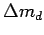From thewe get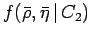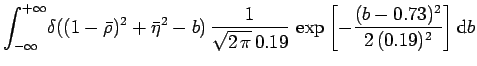(11)(12)

shown by 3-D plots in Fig. 4.

We can already combine the information from the two constraints, just remembering a condition stated in the second paragraph of section 2: In the ideal case we would consider the points of the circle equally likely if there were no other experimental or theoretical a priori information which would lead to assign different weights to the different points. But this is exactly what each partial inference does. Therefore, in order to combine the two constraints we just need to multiply the weights of each point and normalize the distribution: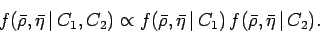(13)

The 3-D plot of the normalized distribution is shown in Fig. 3. We see that with these two constraints only there is still sign ambiguity, and, in particular, the distribution is specular with respect to the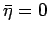axis.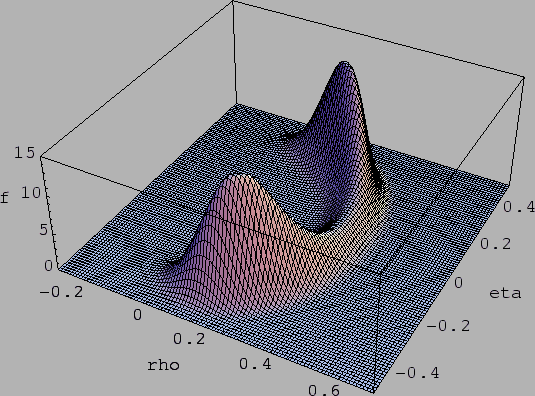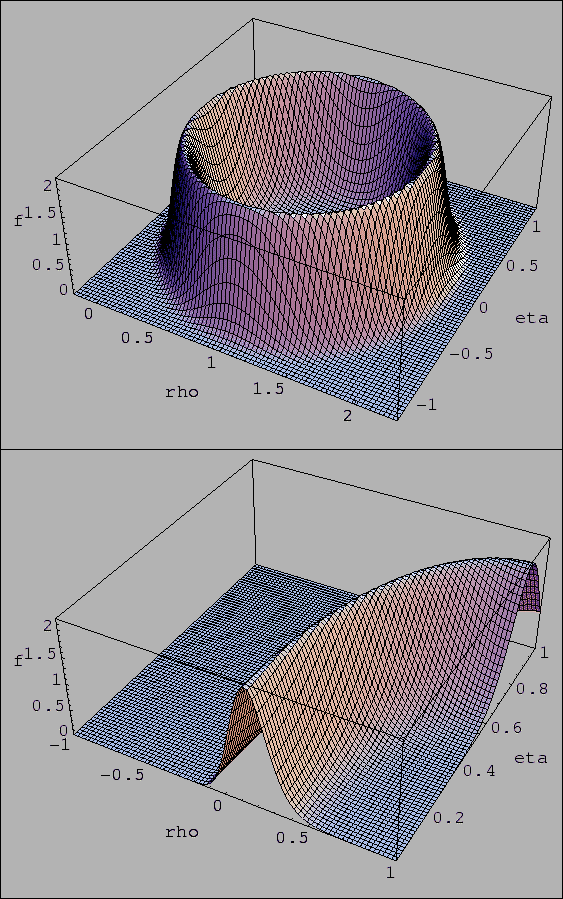Next: Up: Partial results from , Previous:
Giulio D'Agostini 2004-01-20